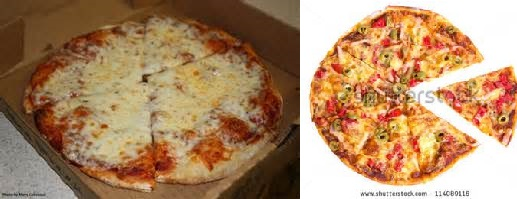Yummy Pizza

Geometry Level 2A pizza shop sells two round pizzas which have same thickness but are different in size. The smaller one has a diameter of 30 cm. The larger one has a diameter of 60 cm and costs twice the price of the smaller one.

In terms of value for money, which of the following statements is true?

Note

• Here we take value to mean price. I.e. which option gets you pizza at the lowest cost per unit area.
×